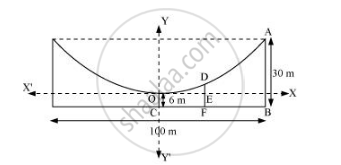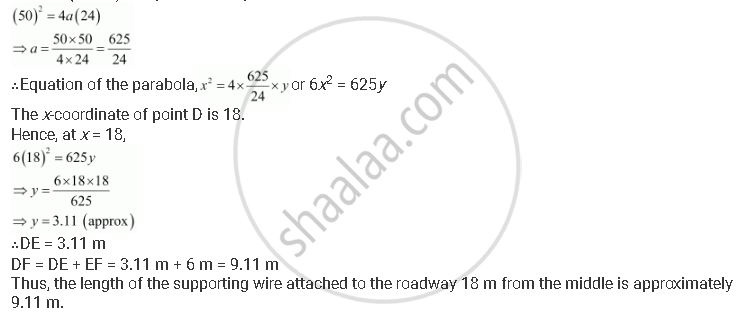# The Cable of a Uniformly Loaded Suspension Bridge Hangs in the Form of a Parabola. the Roadway Which is Horizontal and 100 M Long is Supported by Vertical Wires Attached to the Cable, the Longest Wire Being 30 M and the Shortest Being 6 M. Find the Length of a Supporting Wire Attached to the Roadway 18 M from the Middle. - Mathematics

The cable of a uniformly loaded suspension bridge hangs in the form of a parabola. The roadway which is horizontal and 100 m long is supported by vertical wires attached to the cable, the longest wire being 30 m and the shortest being 6 m. Find the length of a supporting wire attached to the roadway 18 m from the middle.

#### Solution

The vertex is at the lowest point of the cable. The origin of the coordinate plane is taken as the vertex of the parabola, while its vertical axis is taken along the positive y-axis. This can be diagrammatically represented asHere, AB and OC are the longest and the shortest wires, respectively, attached to the cable.

DF is the supporting wire attached to the roadway, 18 m from the middle.

Here, AB = 30 m, OC = 6 m, and BC = 100/2 = 50 m

The equation of the parabola is of the form x2 = 4ay (as it is opening upwards).

The coordinates of point A are (50, 30 – 6) = (50, 24).

Since A (50, 24) is a point on the parabola,Concept: Parabola - Standard Equations of Parabola
Is there an error in this question or solution?

#### APPEARS IN

NCERT Class 11 Mathematics
Chapter 11 Conic Sections
Q 3 | Page 264